# Negative zero leading to unexpected issue in Rule Engine

Hi !

I’m having an issue while manipulating zero, math formula and rule engine. I’ve created a basic worflow as demo. My issue is that multiplying a zero with a negative value creates a negative zero. Which is then no more identified as zero by the Rule Engine…

The workflow here :Give true for one :

and false for the other :

Here is the complete workflow : demo_negative_zero.knwf (9.2 KB)

How should I tackle this ?

Thanks,

Thomas

1 Like

Hello @ThomasLLN,

either in Math Formula with if() function:
`if(\$value\$==0,\$value\$,\$value\$*-1 )`

Either in Rule Engine with checking for -0:
`\$value\$ = 0 OR \$value\$ = -0 => TRUE`

Would that work?

Br,
Ivan

1 Like

Hi @ipazin ,

Neither of those are working. But this gave me the tips to write this in the Rule Engine :

`\$value\$ = 0.0 OR \$value\$ = -0.0 => TRUE`

And this is working. It’s still a strange behaviour. I should not have to check for both sign.

Thanks !

T.

1 Like

Wow, this is quite a pitfall… also the Row Filter differentiates between positive and negative zero.

In: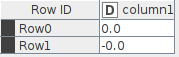Row filter: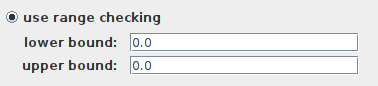Out: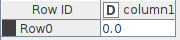Instead, in order to catch both rows one has to do this: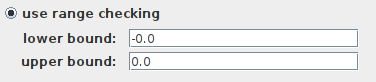This is a major “gotcha” that 99% of the users are probably not aware of… I certainly wasn’t.

Strangely, the Numeric Row Splitter node sees positive and negative zero as the same.

Best
Aswin

As a general rule in informatics, a double should never be compared using an “=” condition, because double numbers are coded in a way (using floating comma) that leads often to rounding problems with decimals and hence wrong comparisons.

The right way to overcome this kind of problems is to set a condition which integrates the rounding error, for instance in the rule engine:

value < 0.00000001 AND value > -0.00000001 => “It is a Zero coded as double”
TRUE => “It is not Zero”

Up to you to define which is your acceptable rounding value (here set to 0.00000001) to decide when a -double coded number- should be considered as equal to Zero.

Hope this helps.

Best

Ael

1 Like

Hi @aworker ,

Thanks for the head up. I’m fully aware of this limitation. In my specific contexte, I’m searching for true 0 coded as double. And now I have to search for 0.0 and -0.0. Not that a big issue, but that’s something that you need to know…

Best

T.

1 Like

I fully agree with you. May be the problem you have spotted is related to the way KNIME converts strings into doubles in some nodes. A similar problem is described in the post below when rounding integers:

The problem may thus come from the fact that the numbers one enters in the Table Creator are originally strings that are converted into doubles. I guess the String to double conversion done by the -Table Creator- node is not handled in the same way for a 0.0 and a -0.0, most probably because of the rounding scheme used by KNIME to convert strings into doubles.

Best

Ael

3 Likes

Hello @ThomasLLN, @Aswin and @aworker,

this seems like a good explanation:

Simple solution to avoid -0.0 is to add 0.0.

Br,
Ivan

2 Likes

Hi all,

It appears that positive zero double and negative zero double in Java are two different things… but how does this work when someone uses == to compare a positive and a negative zero?

Let’s start with something that is positive absolute zero, no rounding, by having a Math Expression node return a 0: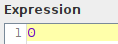Then, in a second Math Expression node, take the negative of this positive zero and put it in a new column: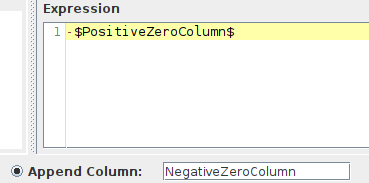Result:Are these the same? Let’s ask a Java Snippet: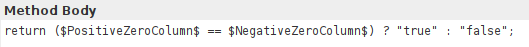Result: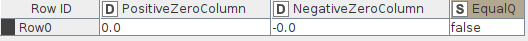So, Java does not regard a table cell with a positive zero as the same as a table cell with a negative zero.

However…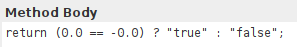yields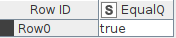Even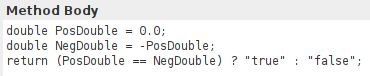yields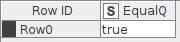That’s odd! Is NegDouble really negative zero here? Yep: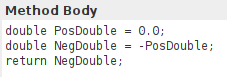I don’t understand… Does it have something to do with Double object-to-primitive unboxing?

Edit:
in the example below i force Java to compare primitives… and now the table cell values are suddenly equal: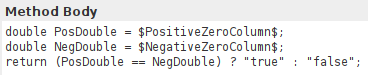2 Likes

@Aswin thanks for highlighting this. Very interesting and important to know.

For completeness, Python does not care about it when converting the KNIME double data into Python (Pandas) double data, as shown below:

Amazing!

1 Like

Neither does R: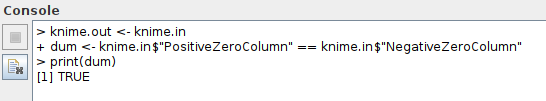But I still don’t fully understand the difference between(yields false)

and(yields true)

I mean, the former is apparently not comparing primitives, but it is also not comparing references. So what does it do?

1 Like